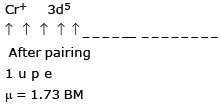Courses

# MCQ (Practice) - Coordination Cmpd (Level 2)

## 25 Questions MCQ Test | MCQ (Practice) - Coordination Cmpd (Level 2)

Description
This mock test of MCQ (Practice) - Coordination Cmpd (Level 2) for Class 12 helps you for every Class 12 entrance exam. This contains 25 Multiple Choice Questions for Class 12 MCQ (Practice) - Coordination Cmpd (Level 2) (mcq) to study with solutions a complete question bank. The solved questions answers in this MCQ (Practice) - Coordination Cmpd (Level 2) quiz give you a good mix of easy questions and tough questions. Class 12 students definitely take this MCQ (Practice) - Coordination Cmpd (Level 2) exercise for a better result in the exam. You can find other MCQ (Practice) - Coordination Cmpd (Level 2) extra questions, long questions & short questions for Class 12 on EduRev as well by searching above.
QUESTION: 1

Solution:

Refer theory

QUESTION: 2

### The complex ion which has no `d' electrons in the central metal atom is;

Solution:

[Co3+(NH3)6]3+ Co3+    d6
[Fe3+(CN)6]3– Fe3+    d5
[Cr3+(H2O)6]3+Cr3+    d3
[Mn7+O4] Mn7+    d0

QUESTION: 3

### Oxidation number of Fe in violet coloured complex Na4[Fe(CN)5(NOS)] is :

Solution:

Let the O. N. of Fe = x
4 + x + (–1) × 5 + (–1) = 0
x = + 2

QUESTION: 4

Complexes [Co(SO4)(NH3)5]Br and [CoBr(NH3)5]SO4 can be distinguished by :

Solution:

Br + Ba2+ → x
SO42– + Ba2+ → BaSO4(s) (white ppt)
Br+ Ag+ → AgBr(s) Pale yellow ppt
SO42– + Ag+ → x
2 - ions form both the complex but magnitude of chene is different, second complex is more electrically conducting.

QUESTION: 5

Amongst the following ions, which one has the highest paramagnetism?

Solution:

[Cr(H2O)6]3+
has maximum 3 unpaired electrons
[Fe(H2O)6]2+
has maximum 4 unpaired electrons
[Zn(H2O)6]2+
has maximum 0 unpaired electrons
[Cu(H2O)6]2+
has maximum 2 unpaired electrons H2O is weak field ligand.

*Multiple options can be correct
QUESTION: 6

Which of the following will produce a white preciptiate upon reacting with AgNO3 ?

Solution:

Complex contains Cl out side the coordination sphere gives white ppt. AgNO3

*Multiple options can be correct
QUESTION: 7

Which isomer of CrCl3.6H2O is dark green in colour and forms one mole of AgCl with excess of AgNO3 solution

Solution:

Complex contains only one Cl out side the coordination sphere which gives one mole white ppt. AgNO3

*Multiple options can be correct
QUESTION: 8

Which of the following are π-bonded organometallic compounds ?

Solution: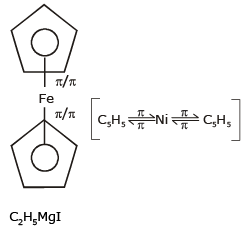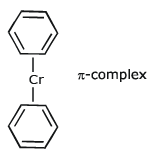*Multiple options can be correct
QUESTION: 9

Which of the following is /are inner orbital complex (es) as well as diamagnetic in nature.

Solution:

[Ir(H2O)6]3+ d2SP3 It is Inner orbital complex, dimagnetic
[Ni (NH3)6]2+ SP3d2 Outer orbital complex, Para Magnetic
[Cr (NH3)6]3+ d2SP3  Inner orbital complex, Para Magnetic
[CO(NH3)6]3+ d2SP3 Inner orbital complex, di Magnetic

*Multiple options can be correct
QUESTION: 10

Which of the following is /are correct about [Cu(NH3)4]SO4

Solution:

[Cu2+(NH3)4] SO4
SO42– +Ba2+ → BaSO4(s)
(White ppt)
Conductivity corresponds to two ions

*Multiple options can be correct
QUESTION: 11

Which of the followng isomerism is /are shown by the complex [CoCl2(OH)2(NH3)2]Br ?

Solution:

[COCl2(OH)2(NH3)2] Br & [CoClBr(OH)2 (NH3)2]Cl are ionisation isomers.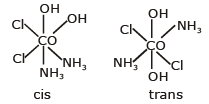NO POS so optically active

*Multiple options can be correct
QUESTION: 12

Both geometrical and optical isomerism are shown by

Solution: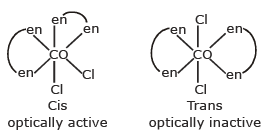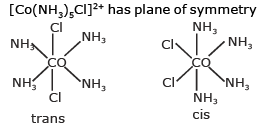both are optically inactive
[CO(OX)3]3– No geometrical isomers only optical isomers

*Multiple options can be correct
QUESTION: 13

WHich of the following complexes have tetrahedral shape ?

Solution:

[Cu(NH3)4]2+ dsp2 square planner paramagnetic
[Ni(CO)4] sp3 Tetrahedral Dimagnetic
[Ni(CO)4] sp3 Tetrahedral Dimagnetic
[Zn(NH3)]42+sp3 Tetrahedral Dimagnetic

*Multiple options can be correct
QUESTION: 14

Which of the following is /are paramagnetic

Solution:

[Ru (H2O)6]3+ d2sp3 1 unpaired electron (upe) octahedral
[Mn(CO)s] dsp3 0 upe TBP
[Fe(NH3)6]2+ sp3d24 upe octahedral
Cr2O72– sp3 0 upe dimagnetic

*Multiple options can be correct
QUESTION: 15

Co-ordination number of Cr in CrCl3.5H2O is six. The volume of 0.1 N AgNO3needed to ppt. the chlorine in outer sphere in 200 ml of 0.01 M solution of the complex is /are :

Solution:

Applying law of equivalence eq of complex = eq of Ag NO3
[Cr(H2O)5Cl]Cl2
200×0.01×2=0.1×v
= 40 mL
[Cr(H2O)4Cl2]Cl. H2O
200 × 0.01 × 1 = 0.1 xV
V = 20 ml

*Multiple options can be correct
QUESTION: 16

When a transition metal ion (usually) is involved in octahedral complex formation, the five degenerate d-orbitals split into two set of degenerate orbitals (3 + 2). Three degenerate orbitals of lower energy (dxy, dyz, dzx) and a set of degenerate orbitals of higher energy (dx2 – y2 and dz2). The orbitals with lower energy are called t2g orbitals and those with higher energy are called eg orbitals.

In octahedral complexes, positive metal ion may be considered to be present at the centre and negative ligands at the corner of a regular octahedron. As lobes of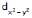and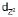lie along the axes, i.e., along the ligands the repulsions are more and so high is the energy. The lobes of the remaining three d-orbitals lie between the axes. i.e., between the ligands. The repulsion between them are less, so lesser the energy. In the octahedral complexes, if metal ion has electrons more than 3 then for pairing them the option are

(ii) Pairing may start normally with 6th electrons when t2g and eg orbitals are singly filled.

Q.

In which of the following configuration hybridisation and magnetic moment of octahedral complexes are independent of nature of ligands.

(i) d3 configuration of any metal cation.
(ii) d6 configuration of IIIrd transition series metal cation.
(iii) d8 configuration of Ist transition series metal cation.
(iv) d7 configuration of any metal cation

Select the correct code :

Solution:
*Multiple options can be correct
QUESTION: 17

When a transition metal ion (usually) is involved in octahedral complex formation, the five degenerate d-orbitals split into two set of degenerate orbitals (3 + 2). Three degenerate orbitals of lower energy (dxy, dyz, dzx) and a set of degenerate orbitals of higher energy (dx2 – y2 and dz2). The orbitals with lower energy are called t2g orbitals and those with higher energy are called eg orbitals.

In octahedral complexes, positive metal ion may be considered to be present at the centre and negative ligands at the corner of a regular octahedron. As lobes ofandlie along the axes, i.e., along the ligands the repulsions are more and so high is the energy. The lobes of the remaining three d-orbitals lie between the axes. i.e., between the ligands. The repulsion between them are less, so lesser the energy. In the octahedral complexes, if metal ion has electrons more than 3 then for pairing them the option are

(ii) Pairing may start normally with 6th electrons when t2g and eg orbitals are singly filled.

Q.

Which of the following electronic arrangement is /are possible for inner orbital oct complex.

(I)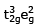(II)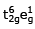(III)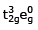(IV)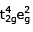Select the correct code :

Solution:

t32ge2g  outer orbital
t32ge0g  inner orbital
t42ge2g  outer orbital
t2g6e1g  inner  orbital by excitation of electron.

*Multiple options can be correct
QUESTION: 18

When a transition metal ion (usually) is involved in octahedral complex formation, the five degenerate d-orbitals split into two set of degenerate orbitals (3 + 2). Three degenerate orbitals of lower energy (dxy, dyz, dzx) and a set of degenerate orbitals of higher energy (dx2 – y2 and dz2). The orbitals with lower energy are called t2g orbitals and those with higher energy are called eg orbitals.

In octahedral complexes, positive metal ion may be considered to be present at the centre and negative ligands at the corner of a regular octahedron. As lobes ofandlie along the axes, i.e., along the ligands the repulsions are more and so high is the energy. The lobes of the remaining three d-orbitals lie between the axes. i.e., between the ligands. The repulsion between them are less, so lesser the energy. In the octahedral complexes, if metal ion has electrons more than 3 then for pairing them the option are

(ii) Pairing may start normally with 6th electrons when t2g and eg orbitals are singly filled.

Q.

Select incorrect match for the following complexes.

Solution:

[Co(H2O)6]3+ Δ> P as for
Co3+  Cl, I, Br, F are only weak field ligands, rest are strong field ligands.

QUESTION: 19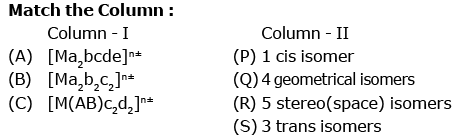(where AB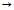Unsym. bidentate ligand, a,b,c,d & emonodentate ligands)

Solution:

(A)–S ; (B)–P ; (C)–Q

QUESTION: 20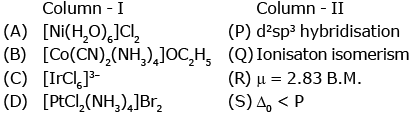Solution:

(A)–R,S ;  (B)–P,Q ;  (C)–P ;  (D)–P,Q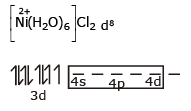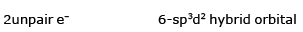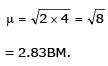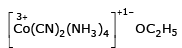CN & NH3 are strong field ligand
CO3+d6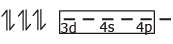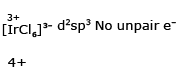[PtCl2(NH3)4]Br2  d2sp3 No unpair e

QUESTION: 21

The pair of compounds having metals in their highest oxidation state is

[JEE-2004]

Solution: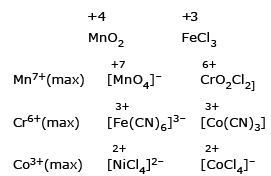QUESTION: 22

The compound having tetrahedral geometry is

Solution:

Cl is weak field ligand & CN is strong field ligand & for transition metal belonging to 2nd & 3rd transition series all lignads acts as strong field ligand.
[Ni(CN)4]2– dsp2 [PdCl4]2– dsp2
[Pd(CN)4]2– dsp2 [NiCl4]2– sp3

QUESTION: 23

Spin only magnetic moment of the compound Hg[Co(SCN)4] is

[JEE-2005]

Solution: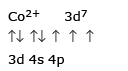3 unpaired electron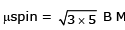4sp3 hybrid orbital =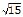QUESTION: 24

Which of the following pair is expected to exhibit same colour in solution ?

Solution:

The chemical unit having same no. of unpaired electron has nearly same colour due to nearly same value of crystal field spliting energy Cu2+ in CuCl2 V4+ in VOCl2 has one unpaired electron each.

QUESTION: 25

Which type of isomerism is shown by [Co(NH3)4Br2] Cl ?

Solution: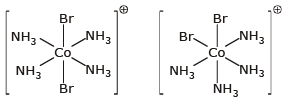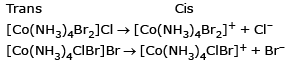n = 1
O.N. of Cr in the complex